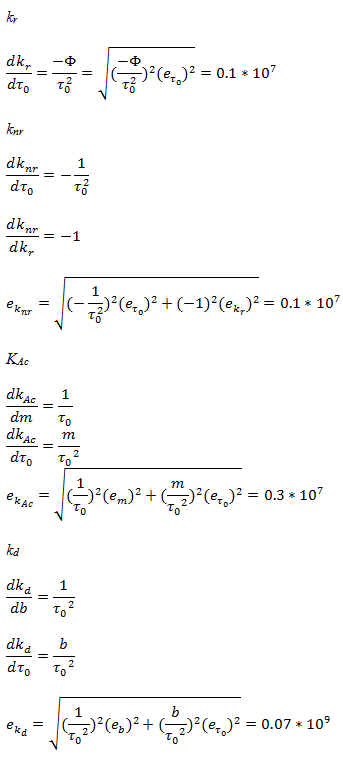# Determining Rate Constants of 2-Naphthol in Varying pH Solutions Using Fluorescence

Abstract:

Rate constants are important to quantify the rate of a chemical reaction. The protonation and deprotonation rate constants were determined of the lowest excited singlet state of 2-naphthol (ArOH) in aqueous solution. The rate constants were determined by measuring the fluorescence intensity, If, proportional to [ArOH*] at acidic, basic, and varying ammonium acetate (NH4Ac) concentrations (0.01-0.11M). Radiative, kr, and nonradiative, knr, were calculated to be (2.6±0.1)*107 s-1 and (1.18±0.7)*108 s-1 using literature values for fluorescence quantum efficiency, Φ=0.18 and the natural fluorescence,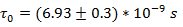. Rate constants for unassisted, kd, and acetate assisted, kAc, deprotonation were determined by a Stern-Volmer plot, intensity as a function of the [NH4Ac], to be (8.1±0.3)*107 s-1 and (2.56±0.07)*109 M-1 s-1, respectively. Protonation constant, kp, was determined to be (6.19±0.2)*1010 M-1 s-1 using the literature excited state acidity constant Ka*= 0.00131. Nonradiative decay occurred at a faster rate than radiative decay and acetate assisted deprotonation occurred at a faster rate than unassisted. Using the Stokes-Einstein-Smolouchowski equation kdiff was calculated to be 7.42*109 dm3mol-1s-1. Both kdiff and kAc have the same order of magnitude signifying kAc is dependent on diffusion. Rate constant results corresponded with literature values with a percent difference of 0.9%, 30%, and 11% for kr+ knr, kd, and kp, respectively.

Experimental:

A series of five aqueous solutions in 25-mL volumetric flasks with varying NH4Ac concentration between 0.010 and 0.10 M and ArOH stock solution (4.0*10-4 M) were prepared. Two solutions were made with stock solution of ~0.1 M NaOH and ~0.1M H2SO4 to observe fluorescence in acid and base. Reagents used in Table 1 and amount stock solution used for each solution in Table 5 under appendix. Deionized water was used as a blank and fluorescence measurements were taken with a Photon Technology International fluorometer with FeliX32 analysis module computer program at excitation wavelength of 320 nm in the emission range of 330 to 600 nm.

Table 1. Reagents used for stock solution in 250 mL volumetric flasks.

 Reagent Formula Molecular Weight (g mol-1) Density (g mL-1) CAS Number Stock Concentration (M) Sodium Hydroxide NaOH 40.00 2.13 1310-73-2 0.10924±0.000005 Ammonium Acetate NH4Ac 77.08 1.073 631-61-8 0.11514±0.00006 Sulfuric Acid H2SO4 98.079 1.84 7664-93-9 0.1126±0.0002 2-Naphthol C10H7OH 144.17 1.22 135-19-3 0.00042±0.0000

Data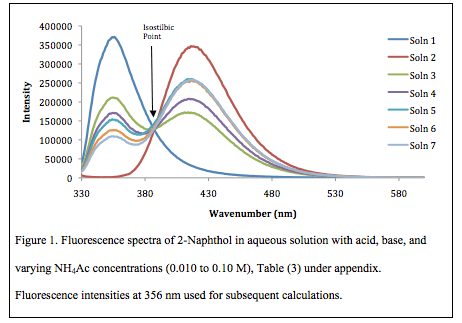Table 2. Acetate concentration in each solution with the Fluorescence intensity, If, found in Figure 1 at 356 nm.

 Solution [Ac–], M If ,,𝑰–𝒇–𝟎.-,𝑰–𝒇..− 𝟏 3 0.0099 210591 0.7619 4 0.0299 170159 1.1805 5 0.0598 153216 1.4216 6 0.0801 124891 1.9709 7 0.0990 108405 2.4227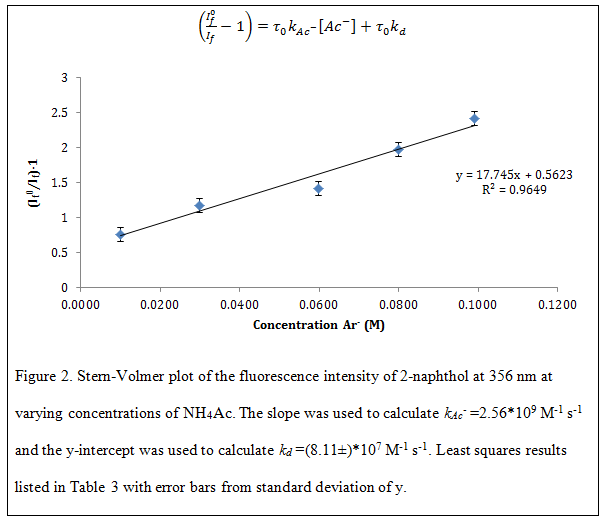Table 3. Least squares results from Figure 2, Stern-Volmer plot of 2-naphthol fluorescence.

 LINEST RESULTS Slope (m) 18.0 0.6 y-intercept (b) Standard Deviation of Slope (Sm) 2.0 0.1 Standard Deviation of y-intercept (Sb) Linear Regression (R2) 0.9649 0.1 Standard Deviation of y (Sy)

Table 4. Rate constants determined compared to literature values using the same method as this experiment with percent difference.

 Rate Constant Experimental Literature3 Percent Difference kr (s-1) (2.6±0.1)*107 kr+knr=1.43*108 0.9% knr (s-1) (1.18±0.07)*108 kd (s-1) (8.1±0.3)*107 6.0*107 29% kAc– (M-1 s-1) (2.56±0.07)*109 2.3*109 11% kp (M-1 s-1) (6.2±0.2)*1010 1.9*1011 102% kdiff (M-1 s-1) 7.42*109 – –

Note: Large percent difference for kp associated with using different Ka*

Conclusion

The protonation and deprotonation rate constants of 2-naphthol in the lowest excited singlet state in a range of acidic to basic pH solutions was determined by fluorescence study and Stern-Volmer plot,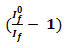as a function of [Ac], Figure 2. The maximum emission intensity for acidic solution was determined to be at 356 nm, 420 nm for basic solution, and the isostilbic point at 387 nm, Figure 1. Fluorescence at 356 was highest in acidic solution due to deprotonation being suppressed1. The rate of acetate-assisted deprotonation was determined to be faster than unassisted with kAc= (2.56±0.07)*109 M-1 s-1 and kd= (8.1±0.3)*107. Nonradiative decay occurred faster than radiative decay due to low reaction energy barrier at rates of knr= (1.18±0.07)*108 s-1 and kr= (2.6±0.1)*107 s-1. The diffusion rate constant, calculated from the Stokes-Einstein-Smolouchowski, was found to be 7.42*109 M-1 s-1 indicating two neutral species due to kdiff same order of magnitude as kAc1. The percent difference for the rate constant is 0.9%, 30%, and 11% for kr+ knr, kd, and kp, respectively. Difference between theoretical and experimental could be due to temperature differences while running the reactions. Determining the rate constants is important to understand reactions under variable conditions.

References

 M. Halpern and G.C. McBane, Experimental Physical Chemistry, 3rd ed., W.H. Freedman and Company, New York, 2006. Experiment 34.

 Atkins, P., J. De Paula “Physical Chemistry”, 9th ed., W. H. Freeman, New York (2010)

 R. Boyer, G. Deckey, C. Marzzacco, M. Mulvaney, C. Schwab, and A. M. Halpern, J. Chem. Educ., 62, 630 (1985).

 Kestin, J.; Sokolov, M.; Wakeham, W. A. Viscosity of Liquid Water in the Range -8°C to 150°C. J. Phys. Chem. 7, 941 (1978).

 T. Forster, Z. Elektrochem., 54, 42 (1950).

 Smith, J.; Fluorescence Decay of 2-Naphthol, Handout, Print.

Appendix
Table 5. Amount of stock solution used to make solutions that were analyzed by the fluorometer.

 Analyte Soln. 1 (Acidic) Soln. 2 (Basic) Soln. 3 (Buffer 1) Soln. 4 (Buffer 2) Soln. 5 (Buffer 3) Soln. 6 (Buffer 4) Soln. 7 (Buffer 5) Soln. 8 (Blank) ArOH 2.5 mL 2.5 mL 2.5 mL 2.5 mL 2.5 mL 2.5 mL 2.5 mL 2.5 mL H2SO4 22.5 mL — — — — — — — NaOH — 22.5 mL — — — — — — NH4Ac 0.01M — — 2.25 mL — — — — — NH4Ac 0.03M — — — 6.80 mL — — — — NH4Ac 0.06 M — — — — 13.6 mL — — — NH4Ac 0.08 M — — — — — 18.2 mL — — NH4Ac 0.11 M — — — — — — 22.5 mL — Deionized water — — 20.25mL 15.7 mL 8.9 mL 4.3 mL — 25 mL Total Volume 25 mL 25 mL 25 mL 25 mL 25 mL 25 mL 25 mL 25 mL

Table 6. Fluorescence Decay for 2-Naphthol in 0.10 M H2SO4 at 25C.

 Time (ns) Intensity (photons emitted nanosecond-1) Natural Log (Intensity) 0 21753 9.988 1 18907 9.847 2 16380 9.704 3 14171 9.559 4 12432 9.428 5 10757 9.283 6 9288 9.136 7 8138 9.004 8 7083 8.865 9 6014 8.709 10 5350 8.585CalculationsFluorescence Quantum Efficiency Ф=0.18The slope (m) of Figure 2 was used to calculateThe y-intercept (b) of Figure 2 was used to calculateError Calculations